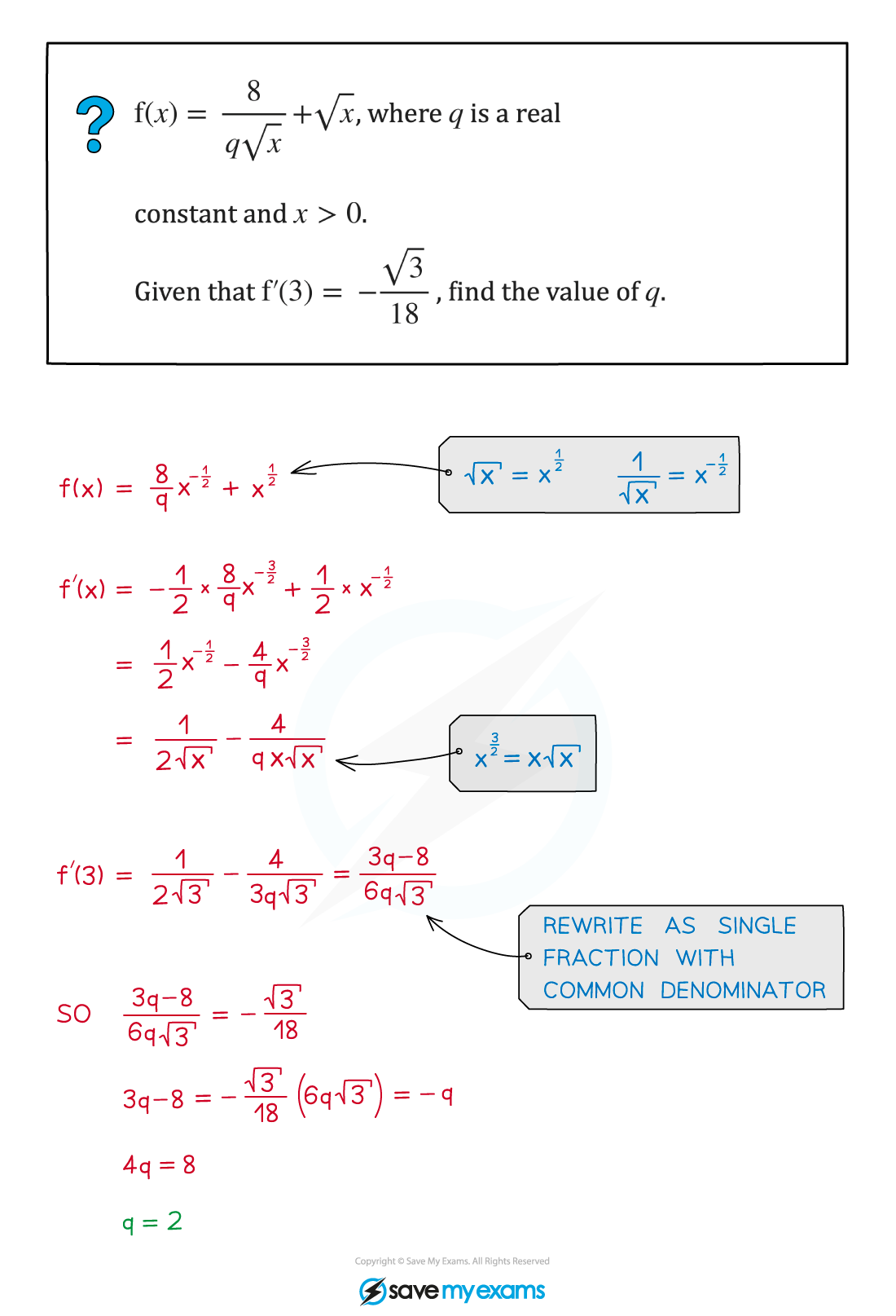# AQA A Level Maths: Pure复习笔记7.1.3 Differentiating Powers of x

### Differentiating Powers of x

#### How do I differentiate expressions involving powers of x?

• Powers of x are differentiated according to the following formulae:• Differentiating terms with positive integer powers of x is straightforward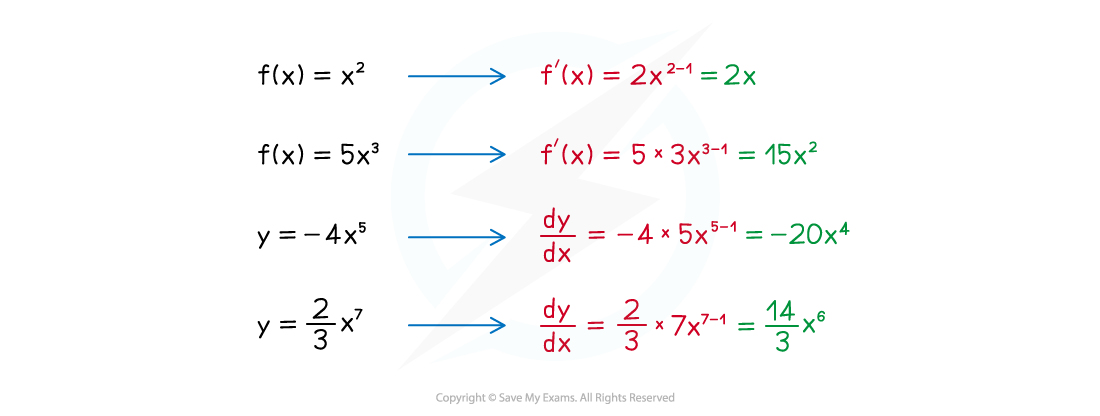• But negative and fractional powers of x can also be differentiated this way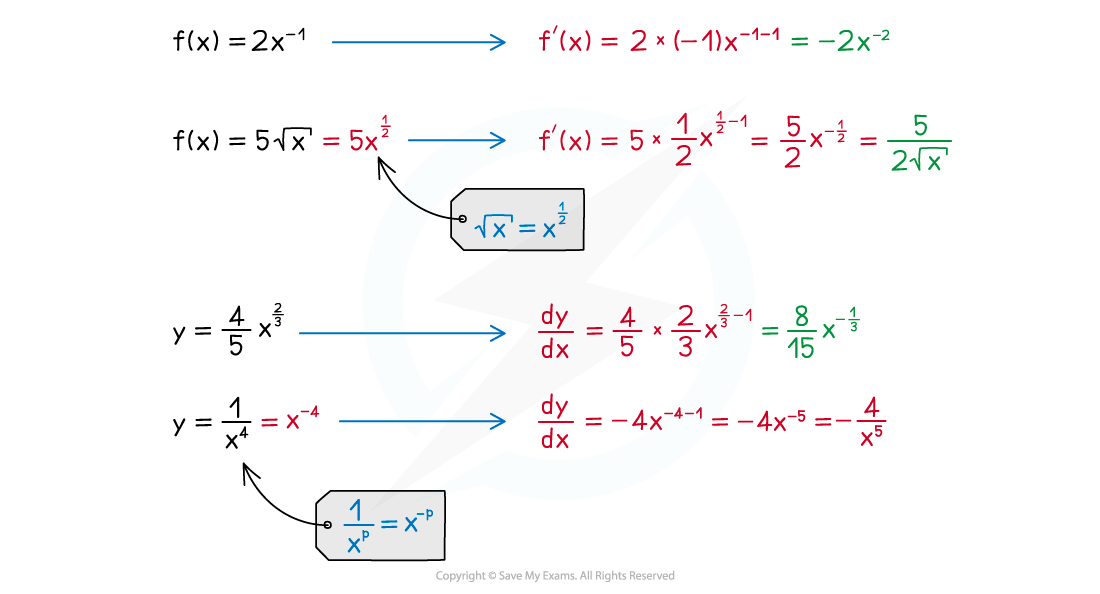• Don't forget these two special cases:• These allow you to differentiate constants and linear terms in x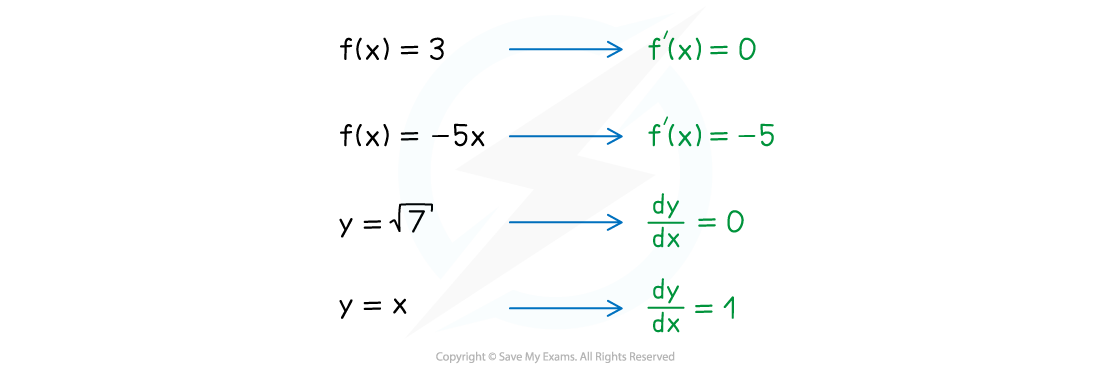• If the expression involves a sum or difference of terms just differentiate one term at a time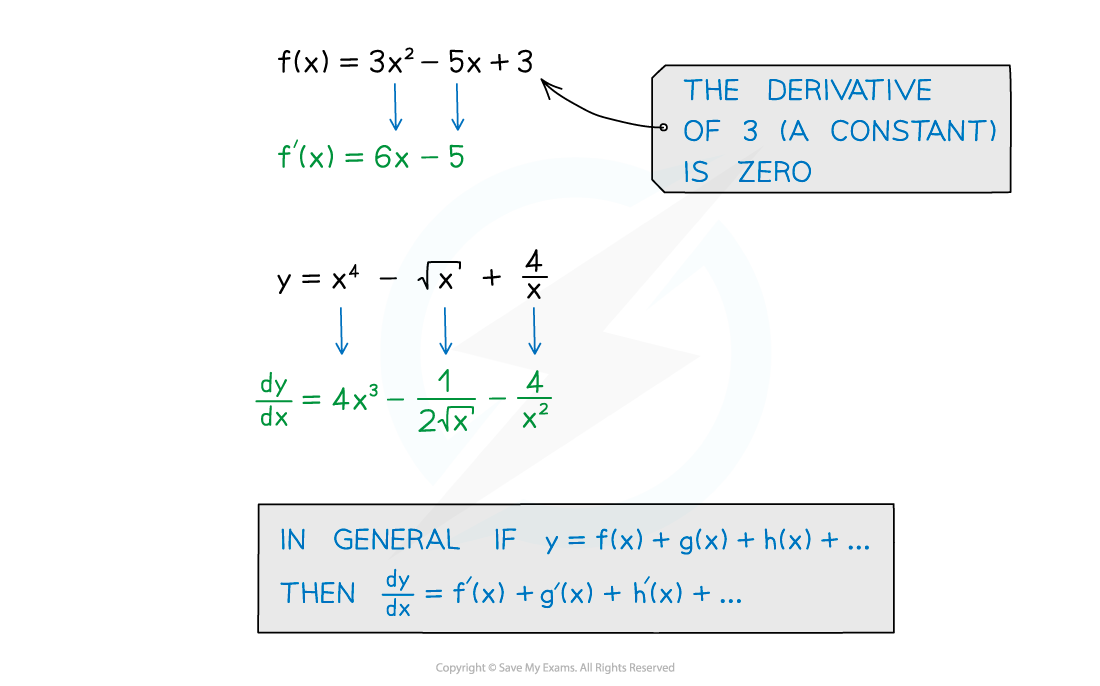#### Exam Tip

• Take extra care when differentiating negative and fractional powers of x as mishandling negative signs and fractions is a common way to lose marks in these sorts of questions.

#### Worked Example